PSABM5UH - Practice---Acc7.5 Lesson 2 Representing Proportional Relationships (7.RP.A.2, 8.EE.B, 8.EE.B.5)

The tortoise and the hare are having a race. After the hare runs 16 miles the tortoise has only run 4 miles. The relationship between the distance x tortoise “runs” in miles for every y miles the hare runs is y = 4x. Graph this relationship.

Take a picture of your graph and upload it using the "insert image" icon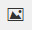. If you can't upload an image, describe in detail what your graph looks like.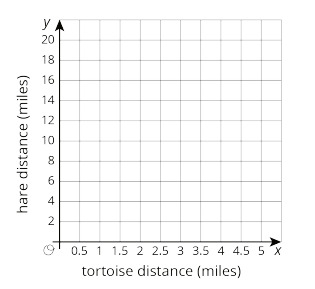Part A)

The table shows a proportional relationship between the weight on a spring scale and the distance the spring has stretched.

Complete the table.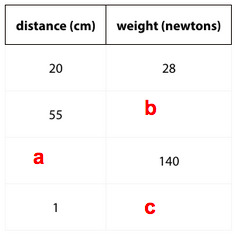a = ________

Type your answer below as a number (example: 5, 3.1, 4 1/2, or 3/2):
Part B)

The table shows a proportional relationship between the weight on a spring scale and the distance the spring has stretched.

Complete the table.b = ________

Type your answer below as a number (example: 5, 3.1, 4 1/2, or 3/2):
Part C)

The table shows a proportional relationship between the weight on a spring scale and the distance the spring has stretched.

Complete the table.c = ________

Part A)

Students are selling raffle tickets for a school fundraiser. They collect \$24 for every 10 raffle tickets they sell.

Suppose M is the amount of money the students collect for selling raffle tickets. Write an equation that reflects the relationship between M and R.

Complete the equation below

M = _______________

Part B)

Label and scale the axes and graph this situation with M on the vertical axis and R on the horizontal axis. Make sure the scale is large enough to see how much they would raise if they sell 1000 tickets.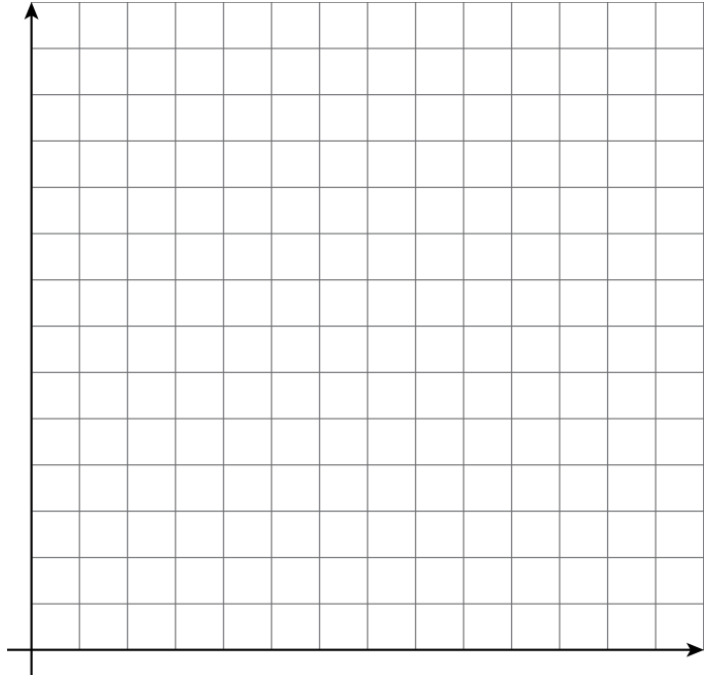Draw your graph on paper, take a picture, and upload it using the image upload icon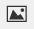If you do not have the ability to upload an image of your work type "Graph is on paper."